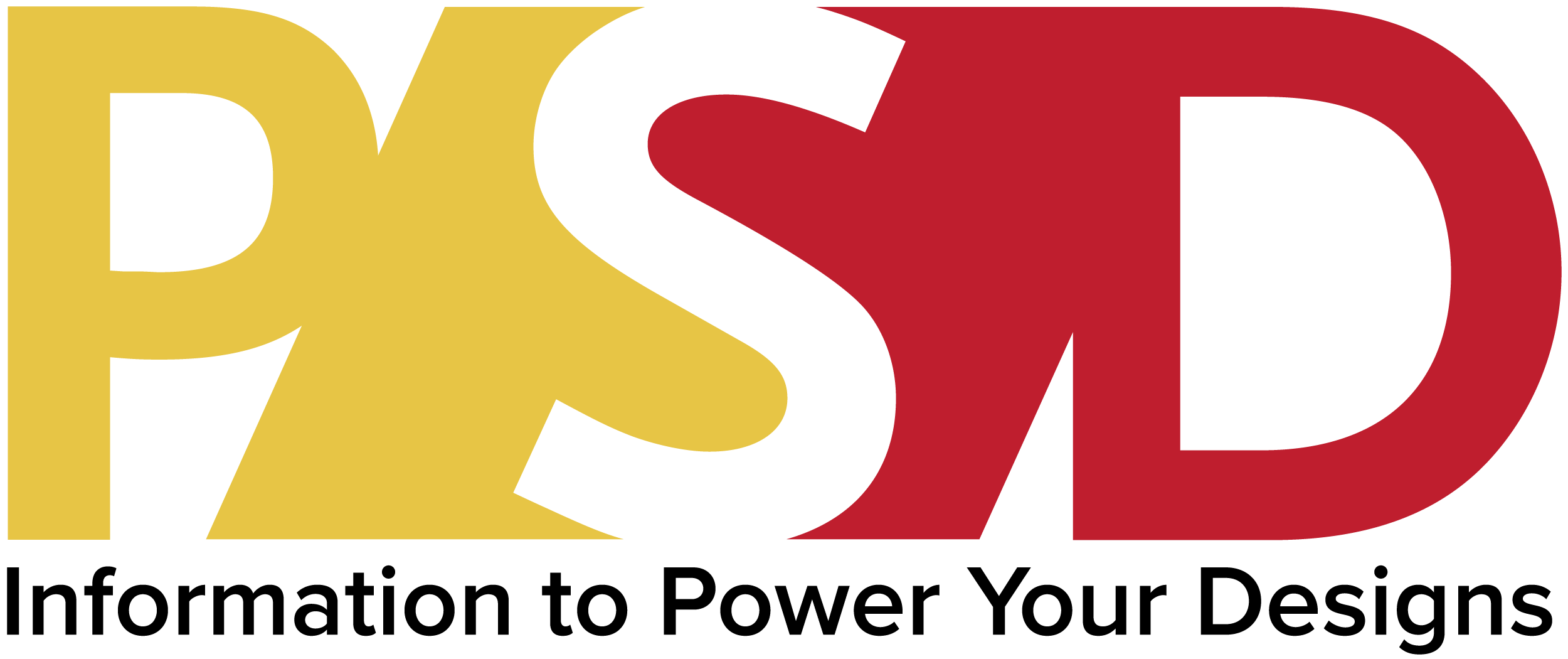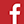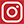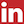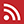﻿

 Product News Industry News Departments Design Centers Multimedia Digital Issues Blogs
 Home > Departments > Design Tips

# Two Steps to High VoltagesPDF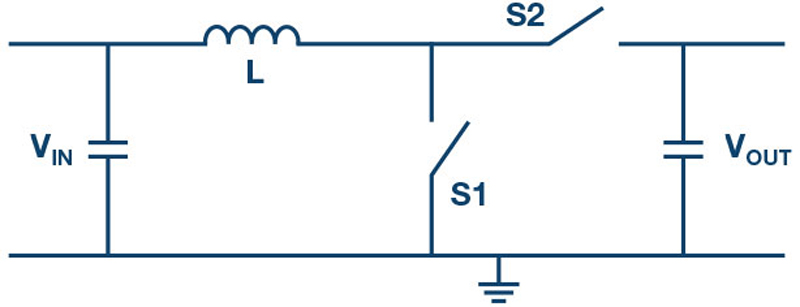Click image to enlarge

Figure 1. Boost topology for generating a high voltage from a low voltage

If a high voltage needs to be generated from a low voltage, a boost converter can be used. This represents one of the three elementary switching regulator topologies and requires only two switches, an inductor, and input and output capacitors. Besides the boost converter, the other basic topologies are the buck converter and the inverting buck-boost converter. Figure 1 shows a schematic of a boost converter. During the on time, switch S1 is closed and energy is stored in coil L. The inductor current increases linearly with the difference between the input voltage and ground potential; that is, with the input voltage. During the offtime, when S1 is open and S2 is closed, the energy stored in the inductor is provided to the output. The voltage across the inductor corresponds in this time period to the output voltage minus the input voltage.

For this interplay to function, there must be enough time both for the charging and for the discharging of the inductor. Through the control loop, this can be imagined as follows: When more energy is required at the output, more energy must be brought to the output from the input. For this, there must be more energy stored temporarily in the inductor. Switch S1 also must conduct for a longer time. However, for a fixed switch frequency, this leads to less time available to get the energy out of the inductor during the off time. Therefore, the output voltage drops below the set target value. This is especially a limitation for the boost topology. Through this, there is a limit to how much above the available input voltage the output voltage can lie. In typical applications, this maximum boost factor lies between 3 and 7.

Click image to enlarge

Figure 2. Maximum possible boost factor determined by inductor resistance DCR (dc resistance)

Figure 2 shows a curve illustrating the typical relationship between the maximum possible boost factor and the respective duty cycle. The exact curve varies according to the relationship between the load resistance at the output of the boost converter and the dc resistance of the inductor. In the diagram shown in Figure 2, a load resistance of 100 Ω has been selected. For an output voltage of 48 V, this corresponds to a loadcurrent of 480 mA. When the series resistance (DCR) of the inductor corresponds to 2Ω, a maximum boost factor of just over 3 would only be possible. For a DCR of 1 Ω, a boost factor slightly higher than 5 could be realized. For higher boost factors, inductors with the lowest possible series resistance values would have to be chosen.

Click image to enalrge

Figure 3. Two-stage concept for generating a very high output voltage from a low input voltage

If a higher boost factor is needed in an application, a two-stage concept is also an option. The new LTC7840  from Analog Devices contains two boost controllers in one chip. This makes it easy to realize two-stage boost concepts. Figure 3 shows an example with a supply voltage of 12V, which is increased to an output voltage of 240V. The two boost stages divide the voltage increase such that each stage only must increase the voltage by a factor of about 4.5.

## Conclusion

This article presented a two-stage concept that makes it possible to achieve much higher boost factors than are achievable with a single stage. Of course, a transformer-based topology can also be selected for significantly stepping up an input voltage. A flyback converter, for example, is popular. However, if galvanic isolation is not necessary, a two-stage boost concept has a few advantages over a flyback converter. There is no need for a large and expensive transformer, as the switching frequencies are not limited by the losses in the transformer core, and the load on the source is continuous and not pulsed. Thus, a two-stage boost concept should be considered in the selection process for numerous applications.

X